# NAND Gate: What is it? (Working Principle & Circuit Diagram)

Contents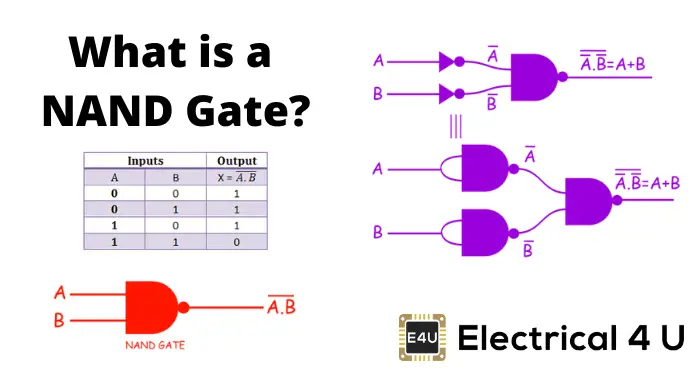## What is a NAND Gate?

A NAND gate (“not AND gate”) is a logic gate that produces a low output (0) only if all its inputs are true, and high output (1) otherwise. Hence the NAND gate is the inverse of an AND gate, and its circuit is produced by connecting an AND gate to a NOT gate. Just like an AND gate, a NAND gate may have any number of input probes but only one output probe.

The NAND gate performs the logical NAND operation. NAND gates are known as universal gates (along with NOR gates), which means they are a type of logic gate which can implement any Boolean function without the need to use any other gate type.

The basic logical construction of the NAND gate is shown below (you can see it is an AND gate followed by a NOT gate):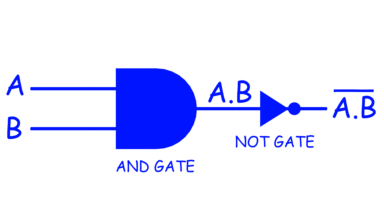The symbol of a NAND gate is similar to the AND gate, but a bubble is drawn at the output point of the AND gate. The symbol of the NAND gate is shown below.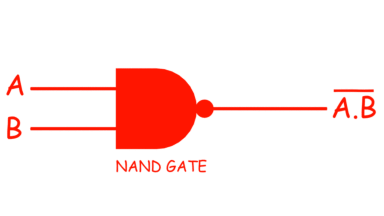In digital electronics, other logic gates include NOT gates, OR gates, NOR gates, XOR gates, XNOR gates.

## NAND Gate Truth Table

NAND gate means “not AND gate”, hence the output of this gate is just reverse of that of a similar AND gate.

We know that the output of the AND gate is only high or 1 when all the inputs are high or 1. In all other cases, the output of the AND gate is low or 0.

In the NAND, the fact is the opposite, here, the output is only logical 0 when and only when all inputs of the gate are 1s, and in all other cases, the output of the NAND gate is high or 1.

Hence, the truth table of a 2 input NAND gate can be represented as: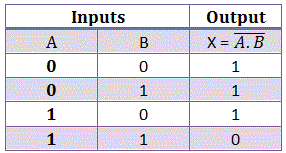You can see that this is just the reverse of the truth table of an AND gate. The truth table of an AND gate is given below for reference: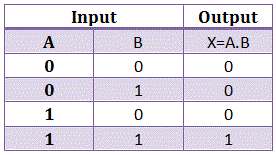Like AND gate a NAND gate can also be more than two inputs, like 3, 4, input NAND gate.
A NAND gate is also referred to as a universal logic gate as all the binary operations can be realized by using only NAND gates.

There are three basic binary operations, AND, OR and NOT. By these three basic operations, one can realize all complex binary operations. Now, we will show how we can achieve all these three binary operations by using only NAND gates.

## How To Make a NOT gate from NAND gates

We can make a NOT gate from a NAND gate.

When both inputs of a two inputs NAND gate are zero, the output is 1, and both inputs of the NAND gate are 1, the output is 0. Hence a NOT gate can very easily be realized from NAND gates just by applying common inputs to the NAND gate or by short-circuiting all the inputs terminals of a NAND gate.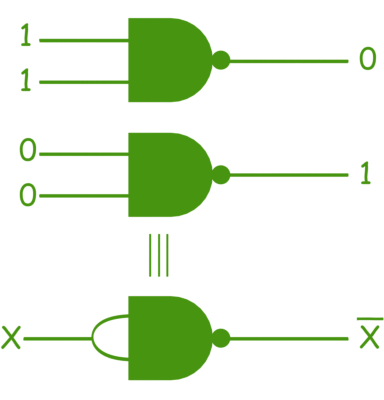Where X is either 1 or 0.

## How To Make an AND gate from NAND gates

We can also make an AND gate from a NAND gate.

As mentioned earlier, a NAND gate is a NOT gate followed by an AND gate, so if we can cancel the effect of NOT gate in a NAND gate, it will become an AND gate. Hence, a NOT gate followed by a NAND gate realizes an AND gate.

In this case, we use the NOT gates realized from NAND gates, and we are showing the logic circuit below: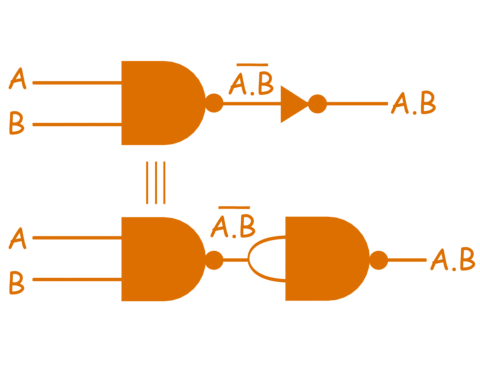## How To Make an OR gate from NAND gates

We can also make an OR gate from a NAND gate.

From De Morgan Theorem we know,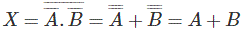The above equation is a logical OR operation.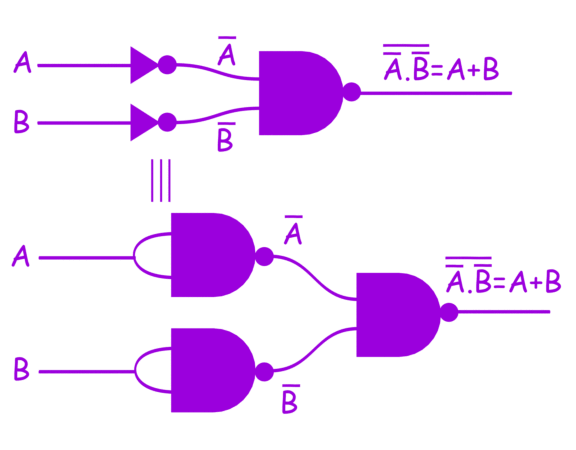The above logic equation can be represented by gates as shown above, where inputs first inverted then passed through a third NAND gate.

The truth table of such circuit is,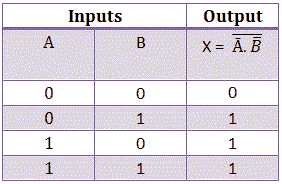Now, we have proved that all three basic binary operations can be realized by using only NAND gates.

Hence, any other simple or complex binary operation must also be realized by using only NAND gates, and hence it is justified to call NAND gates a universal gate.

Want To Learn Faster? 🎓
Get electrical articles delivered to your inbox every week.
No credit card required—it’s 100% free.